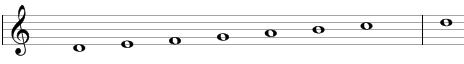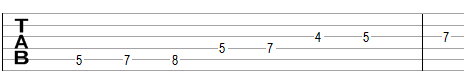# Dorian scale

The Dorian scale is a primary heptatonic scale that can be built using the following steps between notes: 1, ½, 1, 1, 1, ½, 1.

One example of this scale is D, E, F, G, A, B, C. This example is shown below in traditional notation and in guitar tablature notation.This scale is the second mode of the standard major scale or the fourth mode of the natural minor scale. It is the only mode of these scales that is symmetric going up and down.

The Dorian scale is a "primary" heptatonic scale (heptonia prima) as the intervals between any two adjacent notes are either one or two semitones (1 step or ½ steps) and as the two single-semitone intervals are spaced as evenly as possible (going up from the single-semitone interval between E and F we will find three two-semitone intervals – between F and G, G and A, and A and B – before we get to the second single semitone interval between B and C; going in the opposite direction from the single-semitone interval between E and F we will encounter two two-semitone intervals – between D and E and C and D – before we reach again the single-semitone interval between B and C).

## Modes of the Dorian scale

The following are modes of the Dorian scale.

1. Dorian, with steps 1, ½, 1, 1, 1, ½, 1 (D, E, F, G, A, B, C in the example above).
2. Phrygian, with steps ½, 1, 1, 1, ½, 1, 1 (E, F, G, A, B, C, D).
3. Lydian, with steps 1, 1, 1, ½, 1, 1, ½ (F, G, A, B, C, D, E).
4. Mixolydian, with steps 1, 1, ½, 1, 1, ½, 1 (G, A, B, C, D, E, F).
5. Aeolian, also called natural minor, melodic descending minor, or simply minor, with steps 1, ½, 1, 1, ½, 1, 1 (A, B, C, D, E, F, G).
6. Locrian, with steps ½, 1, 1, ½, 1, 1, 1 (B, C, D, E, F, G, A).
7. Ionian or major, with steps 1, 1, ½, 1, 1, 1, ½ (C, D, E, F, G, A, B).

## Three-note chords on the Dorian scale

The following are common triads built on the notes of the Dorian scale.

• On the root of the scale (on the tonic): minor chord (e.g., Dm composed of D, F, A) or suspended chords (Dsus4 = D, G, A and Dsus2 = D, E, A).
• On the second note (on the supertonic): minor chord (Em = E, G, B) or suspended chord (Esus4 = E, A, B).
• On the third note (on the mediant): major chord (F = F, A, C) or suspended chord (Fsus2 = F, G, C).
• On the fourth note (on the subdominant): major chord (G = G, B, D) or suspended chords (Gsus4 = G, C, D and Gsus2 = G, A, D).
• On the fifth note (on the dominant): minor chord (Am = A, C, E) or suspended chords (Asus4 = A, D, E and Asus2 = A, B, E).
• On the sixth note (on the submediant): diminished chord (Bdim = B, D, F).
• On the seventh note (on the leading tone): major chord (C = C, E, G) or suspended chords (Csus4 = C, F, G and Csus2 = C, D, G).

## Four-note chords on the Dorian scale

The following are seventh chords built on the notes of the Dorian scale.

• On the first note: minor seventh chord (e.g. Dmin7 composed of D, F, A, C).
• On the second note: minor seventh chord (Em7 = E, G, B, D).
• On the third note: major seventh chord (Fmaj7 = F, A, C, E).
• On the fourth note: dominant seventh chord (G7 = G, B, D, F).
• On the fifth note: minor seventh chord (Amin7 = A, C, E, G).
• On the sixth note: half-diminished seventh chord (minor seventh chord with a flat fifth, Bm7b5 = B, D, F, A).
• On the seventh note: major seventh chord (Cmaj7 = C, E, G, B).

## Intervals on the Dorian scale

The Dorian scale is composed of the following intervals.

• A major second, e.g., the interval between D and E is equal to two semitones.
• A minor third, e.g., the interval between D and F is equal to three semitones.
• A perfect fourth, e.g., the interval between D and G is equal to five semitones.
• A perfect fifth, e.g., the interval between D and A is equal to seven semitones.
• A major sixth, e.g., the interval between D and B is equal to nine semitones.
• A minor seventh, e.g., the interval between D and C is equal to ten semitones.

Scale, Scale (index)

### Filtered HTML

• Freelinking helps you easily create HTML links. Links take the form of [[indicator:target|Title]]. By default (no indicator): Click to view a local node.